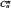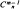# P-Vector

Also found in: Wikipedia.

## P-Vector

a tensor that is skew-symmetric with respect to any two of its indices. It thus is a tensor with either only covariant indices (subscripts) or only contravariant indices (superscripts), where each index can take on values from 1 to n. Moreover, a component of a p-vector changes sign when any two of the component’s indices are interchanged.

If the degree—that is, the number of indices—of a p-vector is equal to 2, 3…. m, we speak of a 2-vector, 3-vector, …, m-vector, respectively. For example, aij is a covariant 2-vector if aij = — aji, and bjki is a contravariant 3-vector if bijk = —bjik = bjki = —bikj = bkij = —

. If only those comonents of the m-vector

ωi1, i2, …, im

are retained for which i1 < i2 < … < im,essential components will remain.

The components of a p-vector can be arranged in a certain way in the form of a rectangular matrix of n rows and columnswhose rank is called the rank of the p-vector. If a p-vector’s rank is equal to its degree (valency), the p-vector is the exterior product of tensors of degree one and is said to be simple.

References in periodicals archive ?
Therefore, variables that are supposed to influence the shape of the rate should be attached to the p-vector.
if the parameters in the p-vector are greater than zero).
The P-vector algorithm approach has been suggested, which is an LMS-like approximate gradient method.
Site: Follow: Share:
Open / Close